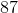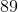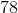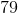# Common Core: 1st Grade Math : Understanding Place Value

## Example Questions

### Example Question #61 : Understanding Place Value

What digit is in the ones place?Explanation:

The ones place is always the first number on the right.

### Example Question #62 : Understanding Place Value

What digit is in the ones place?Explanation:

The ones place is always the first number on the right.

### Example Question #63 : Understanding Place Value

What digit is in the ones place?Explanation:

The ones place is always the first number on the right.

### Example Question #64 : Understanding Place Value

What digit is in the ones place?Explanation:

The ones place is always the first number on the right.

### Example Question #65 : Understanding Place Value

What digit is in the ones place?Explanation:

The ones place is always the first number on the right.

### Example Question #66 : Understanding Place Value

What digit is in the ones place?Explanation:

The ones place is always the first number on the right.

### Example Question #61 : Understanding Place Value

What digit is in the ones place?Explanation:

The ones place is always the first number on the right.

### Example Question #62 : Understanding Place Value

What digit is in the ones place?Explanation:

The ones place is always the first number on the right.

### Example Question #1051 : Common Core Math: Grade 1

What digit is in the ones place?Explanation:

The ones place is always the first number on the right.

### Example Question #67 : Understanding Place Value

What digit is in the ones place?